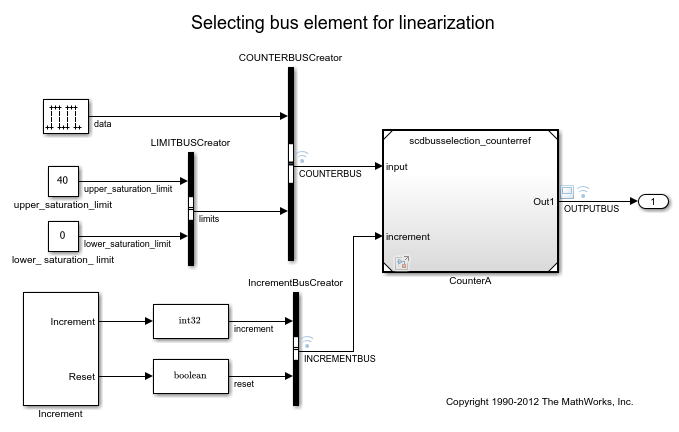Main Content

# linio

Create linear analysis point for Simulink model, Linear Analysis Plots block, or Model Verification block

## Syntax

``io = linio(block,port)``
``io = linio(block,port,type)``
``io = linio(block,port,type,[],busElement)``

## Description

example

````io = linio(block,port)` creates a linearization I/O object that represents an input perturbation analysis point for the signal that originates from the specified output `port` of a Simulink® `block`.```

example

````io = linio(block,port,type)` creates an analysis point of the specified `type`.```

example

````io = linio(block,port,type,[],busElement)` creates an analysis point for an element of a bus signal.```

## Examples

collapse all

Open Simulink model.

```open_system('magball') ```To specify multiple analysis points for linearization, create a vector of linearization I/O objects.

Create an input perturbation analysis point at the output port of the Controller block.

```io(1) = linio('magball/Controller',1); ```

Create an open-loop output analysis point at the output of the Magnetic Ball Plant block. An open-loop output point is an output measurement followed by a loop opening.

```io(2) = linio('magball/Magnetic Ball Plant',1,'openoutput'); ```

View the specified analysis points.

```io ```
```1x2 vector of Linearization IOs: -------------------------- 1. Linearization input perturbation located at the following signal: - Block: magball/Controller - Port: 1 2. Linearization open-loop output located at the following signal: - Block: magball/Magnetic Ball Plant - Port: 1 ```

You can use these analysis points to linearize only the Magnetic Ball Plant subsystem. To do so, pass `io` to the `linearize` command or to an `slLinearizer` interface.

Unlike specifying analysis points directly in the Simulink model, when you create analysis points using `linio`, no annotations are added to the model.

Open Simulink model.

```mdl = 'scdbusselection'; open_system(mdl) ```The `COUNTERBUS` signal, which originates from the COUNTERBUSCreator block, contains multiple bus elements.

Specify the `upper_saturation_limit` bus element as a linearization input. Select this element using dot notation, since it is within the nested `limits` bus.

```io = linio('scdbusselection/COUNTERBUSCreator',1,'input',[],... 'limits.upper_saturation_limit'); ```

## Input Arguments

collapse all

Simulink block from which the analysis point originates, specified as a character vector or string that contains its full block path. For example, to mark an analysis point at an output of the Controller block in the `magball` model, specify `block` as `'magball/Controller'`.

Output port of `block` from which the analysis point originates, specified as a positive integer.

`port` must be a valid port number for the specified `block`.

Analysis point type, specified as one of the following:

• `'input'` — Input perturbation

• `'output'` — Output measurement

• `'loopbreak'` — Loop break

• `'openinput'` — Open-loop input

• `'openoutput'` — Open-loop output

• `'looptransfer'` — Loop transfer

• `'sensitivity'` — Sensitivity

• `'compsensitivity'` — Complementary sensitivity

For more information on analysis point types, see Specify Portion of Model to Linearize.

Bus element name, specified as a character vector or string. When adding elements within a nested bus structure, use dot notation to access the elements of the nested bus. For an example, see Select Individual Bus Element as Analysis Point.

## Output Arguments

collapse all

Analysis point, returned as a linearization I/O object. Use `io` to specify a linearization input, output, or loop opening when using the `linearize` command. For more information, see Specify Portion of Model to Linearize.

Each linearization I/O object has the following properties:

PropertyDescription
`Active`

Flag indicating whether to use the analysis point for linearization, specified as one of the following:

• `'on'` — Use the analysis point for linearization. This value is the default option.

• `'off'` — Do not use the analysis point for linearization. Use this option if you have an existing set of analysis points and you want to linearize a model with a subset of these points.

`Block`

Full block path of the block with which the analysis point is associated, specified as a character vector.

`PortNumber`

Output port with which the analysis point is associated, specified as an integer.

`Type`

Analysis point type, specified as one of the following:

• `'input'` — Input perturbation

• `'output'` — Output measurement

• `'loopbreak'` — Loop break

• `'openinput'` — Open-loop input

• `'openoutput'` — Open-loop output

• `'looptransfer'` — Loop transfer

• `'sensitivity'` — Sensitivity

• `'compsensitivity'` — Complementary sensitivity

For more information on analysis point types, see Specify Portion of Model to Linearize.

`BusElement`

Bus element name with which the analysis point is associated, specified as a character vector or `''` if the analysis point is not a bus element.

`Description`

User-specified description of the analysis point, which you can set for convenience, specified as a character vector.

## Alternative Functionality

### Model Linearizer

You can interactively configure analysis points using the Model Linearizer. For more information see, Specify Portion of Model to Linearize in Model Linearizer.

### Simulink Model

You can also specify analysis points directly in a Simulink model. When you do so, the analysis points are saved within the model. For more information, see Specify Portion of Model to Linearize in Simulink Model.

### `slLinearizer` and `slTuner` Interfaces

If you want to obtain multiple open-loop or closed-loop transfer functions from the linearized system without recompiling the model, you can specify linear analysis points using an `slLinearizer` interface. For more information, see Mark Signals of Interest for Batch Linearization. Similarly, if you want to tune a control system and obtain multiple open-loop or closed-loop transfer functions from the resulting system, you can specify linear analysis points using an `slTuner` interface. For more information, see Mark Signals of Interest for Control System Analysis and Design.

## See Also

### Topics

Introduced before R2006a

## SupportGet trial now